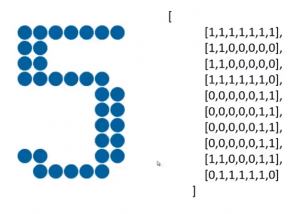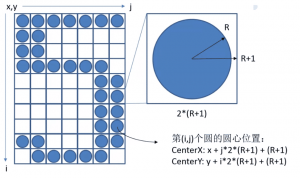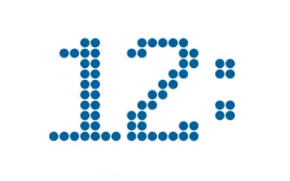## canvas实现绚丽的倒计时效果与动画基础(一)

2018-12-21 分类：前端技术 作者：码云 阅读（6379）

canvas如何实现绚丽的倒计时效果，如下图显示的效果，非常简单，利用之前我为大家整理的canvas绘图和动画基础，你就可以轻松实现这一需求，具体请看《canvas画布绘制动画基础》。倒计时电子钟的实现，通过学习弧线和圆的绘制，进而完成电子钟的绘制效果。

## 倒计时程序基本架构12345678910111213141516171819202122232425262728293031323334353637383940 digit =     [         [             [0,0,1,1,1,0,0],             [0,1,1,0,1,1,0],             [1,1,0,0,0,1,1],             [1,1,0,0,0,1,1],             [1,1,0,0,0,1,1],             [1,1,0,0,0,1,1],             [1,1,0,0,0,1,1],             [1,1,0,0,0,1,1],             [0,1,1,0,1,1,0],             [0,0,1,1,1,0,0]         ],//0         ...         [             [0,1,1,1,1,1,0],             [1,1,0,0,0,1,1],             [1,1,0,0,0,1,1],             [1,1,0,0,0,1,1],             [0,1,1,1,0,1,1],             [0,0,0,0,0,1,1],             [0,0,0,0,0,1,1],             [0,0,0,0,1,1,0],             [0,0,0,1,1,0,0],             [0,1,1,0,0,0,0]         ],//9         [             [0,0,0,0],             [0,0,0,0],             [0,1,1,0],             [0,1,1,0],             [0,0,0,0],             [0,0,0,0],             [0,1,1,0],             [0,1,1,0],             [0,0,0,0],             [0,0,0,0]         ]//:     ];

 12 var canvas = document.getElemntbyId('canvas'); var context = document.getContext('2d');

 12 var WINDOW.WIDTH = 1024; var WINDOW.HEIGHT = 768;

 12 canvas.width = WINDOW.WIDTH; canvas.height = WINDOW.HEIGHT;

 123456 function render(cxt){     var hours = 12;     var minutes = 34;     var seconds = 56;     renderDigit(0, 0, parseInt(hours/10), cxt); }

 1234567891011 function renderDigit (x, y,num, cxt) {     cxt.fillStyle = "rgb(0,102,153)";     for(var i = 0; i < digit[num].length; i++)         for(var j < 0; j < digit[num][i].length; j++)             if(digit[i][j] == 1){                 cxt.beginPath();                 cxt.arc();                 cxt.closePath();                 cxt.fill();             } }

## 倒计时数字钟具体绘制

 12 CenterX:x + j*2*(R+1) + (R+1); CenterY:Y + i*2*(R+1) + (R+1);12345678910111213141516171819202122232425262728293031323334353637383940 var WINDOW_WIDTH = 1024; var WINDOW_HEIGHT = 768; var RADIUS = 8; var MARGIN_TOP = 60; var MARGIN_LEFT = 30; window.onload = function(){     var canvas = document.getElementById('canvas');     var context = canvas.getContext("2d");     canvas.width = WINDOW_WIDTH;     canvas.height = WINDOW_HEIGHT;     render( context ) } function render( cxt ){     var hours = 12     var minutes = 34     var seconds = 56     renderDigit(0, 0, parseInt(hours/10), cxt); } function renderDigit( x , y , num , cxt ){     cxt.fillStyle = "rgb(0,102,153)";     for( var i = 0 ; i < digit[num].length ; i ++ )         for(var j = 0 ; j < digit[num][i].length ; j ++ )             if( digit[num][i][j] == 1 ){                 cxt.beginPath();                 cxt.arc( x+j*2*(RADIUS+1)+(RADIUS+1) , y+i*2*(RADIUS+1)+(RADIUS+1) , RADIUS , 0 , 2*Math.PI )                 cxt.closePath()                 cxt.fill()             } }12 var MARGIN_TOP = 60;//第一个数字距离我们画布上边距的距离 var MARGIN_LEFT = 30;//第一个数字距离我们画布左边距的距离

 1 renderDigit(MARGIN_TOP, MARGIN_LEFT, parseInt(hours/10), cxt);

 12 renderDigit(MARGIN_LEFT, MARGIN_TOP, parseInt(hours/10), cxt); renderDigit(MARGIN_LEFT + 15*(RADIUS + 1), MARGIN_TOP, parseInt(hours%10), cxt);

 1 renderDigit(MARGIN_LEFT + 30*(RADIUS + 1), MARGIN_TOP, 10, cxt);12345678910111213 //时 renderDigit( MARGIN_LEFT , MARGIN_TOP , parseInt(hours/10) , cxt ) renderDigit( MARGIN_LEFT + 15*(RADIUS+1) , MARGIN_TOP , parseInt(hours%10) , cxt ) //冒号 renderDigit( MARGIN_LEFT + 30*(RADIUS + 1) , MARGIN_TOP , 10 , cxt ) //分 renderDigit( MARGIN_LEFT + 39*(RADIUS+1) , MARGIN_TOP , parseInt(minutes/10) , cxt); renderDigit( MARGIN_LEFT + 54*(RADIUS+1) , MARGIN_TOP , parseInt(minutes%10) , cxt); //冒号 renderDigit( MARGIN_LEFT + 69*(RADIUS+1) , MARGIN_TOP , 10 , cxt); //秒 renderDigit( MARGIN_LEFT + 78*(RADIUS+1) , MARGIN_TOP , parseInt(seconds/10) , cxt); renderDigit( MARGIN_LEFT + 93*(RADIUS+1) , MARGIN_TOP , parseInt(seconds%10) , cxt);## 倒计时时间的设置

 1 const endTime = new date(2014,6,11,18,47,52);

（1）在JavaScript中月数这个参数是比较特殊的，它是从0开始一直到11，也就是如果传入的是0就代表着1月，11代表的是12月，这里我想要把这个月份设置成7月那么我传入的就是6，这里大家一定要注意。

（2）大家可能注意到我们demo倒计时呢在消失这个位置只有两位数字，换句话说，一天有24个小时，最多也就是倒计时4天，这是我们程序在设计时的一个限制，如果大家想要打破这个限制，学完这个大家可以将小时扩充到3位数，甚至增加到天的倒计时，月的倒计时，年的倒计时，思路大致一样的，我们的demo暂时设计成这样。所以我在设计日期的时候距离现在不会超过4天。

 1 var ourShowTimeSeconds = 0;

 1234567891011 window.onload = function(){     var canvas = document.getElementById('canvas');     var context = canvas.getContext("2d");     canvas.width = WINDOW_WIDTH;     canvas.height = WINDOW_HEIGHT;     curShowTimeSeconds = getCurrentShowTimeSeconds()     render( context ) }

 1234567 function getCurrentShowTimeSeconds() {     var curTime = new Date();     var ret = endTime.getTime() - curTime.getTime();     ret = Math.round( ret/1000 )     return ret >= 0 ? ret : 0; }

 123456789101112131415 function render( cxt ){     //时、分、秒计算     var hours = parseInt( curShowTimeSeconds / 3600);     var minutes = parseInt( (curShowTimeSeconds - hours * 3600)/60 )     var seconds = curShowTimeSeconds % 60     renderDigit( MARGIN_LEFT , MARGIN_TOP , parseInt(hours/10) , cxt )     renderDigit( MARGIN_LEFT + 15*(RADIUS+1) , MARGIN_TOP , parseInt(hours%10) , cxt )     renderDigit( MARGIN_LEFT + 30*(RADIUS + 1) , MARGIN_TOP , 10 , cxt )     renderDigit( MARGIN_LEFT + 39*(RADIUS+1) , MARGIN_TOP , parseInt(minutes/10) , cxt);     renderDigit( MARGIN_LEFT + 54*(RADIUS+1) , MARGIN_TOP , parseInt(minutes%10) , cxt);     renderDigit( MARGIN_LEFT + 69*(RADIUS+1) , MARGIN_TOP , 10 , cxt);     renderDigit( MARGIN_LEFT + 78*(RADIUS+1) , MARGIN_TOP , parseInt(seconds/10) , cxt);     renderDigit( MARGIN_LEFT + 93*(RADIUS+1) , MARGIN_TOP , parseInt(seconds%10) , cxt); }1234567891011121314151617181920212223242526272829303132333435363738394041424344454647484950515253545556575859 var WINDOW_WIDTH = 1024; var WINDOW_HEIGHT = 768; var RADIUS = 8; var MARGIN_TOP = 60; var MARGIN_LEFT = 30; const endTime = new Date(2014,6,11,18,47,52); var curShowTimeSeconds = 0 window.onload = function(){     var canvas = document.getElementById('canvas');     var context = canvas.getContext("2d");     canvas.width = WINDOW_WIDTH;     canvas.height = WINDOW_HEIGHT;     curShowTimeSeconds = getCurrentShowTimeSeconds()     render( context ) } function getCurrentShowTimeSeconds() {     var curTime = new Date();     var ret = endTime.getTime() - curTime.getTime();     ret = Math.round( ret/1000 )     return ret >= 0 ? ret : 0; } function render( cxt ){     var hours = parseInt( curShowTimeSeconds / 3600);     var minutes = parseInt( (curShowTimeSeconds - hours * 3600)/60 )     var seconds = curShowTimeSeconds % 60     renderDigit( MARGIN_LEFT , MARGIN_TOP , parseInt(hours/10) , cxt )     renderDigit( MARGIN_LEFT + 15*(RADIUS+1) , MARGIN_TOP , parseInt(hours%10) , cxt )     renderDigit( MARGIN_LEFT + 30*(RADIUS + 1) , MARGIN_TOP , 10 , cxt )     renderDigit( MARGIN_LEFT + 39*(RADIUS+1) , MARGIN_TOP , parseInt(minutes/10) , cxt);     renderDigit( MARGIN_LEFT + 54*(RADIUS+1) , MARGIN_TOP , parseInt(minutes%10) , cxt);     renderDigit( MARGIN_LEFT + 69*(RADIUS+1) , MARGIN_TOP , 10 , cxt);     renderDigit( MARGIN_LEFT + 78*(RADIUS+1) , MARGIN_TOP , parseInt(seconds/10) , cxt);     renderDigit( MARGIN_LEFT + 93*(RADIUS+1) , MARGIN_TOP , parseInt(seconds%10) , cxt); } function renderDigit( x , y , num , cxt ){     cxt.fillStyle = "rgb(0,102,153)";     for( var i = 0 ; i < digit[num].length ; i ++ )         for(var j = 0 ; j < digit[num][i].length ; j ++ )             if( digit[num][i][j] == 1 ){                 cxt.beginPath();                 cxt.arc( x+j*2*(RADIUS+1)+(RADIUS+1) , y+i*2*(RADIUS+1)+(RADIUS+1) , RADIUS , 0 , 2*Math.PI )                 cxt.closePath()                 cxt.fill()             } }

「本文为原创文章，版权归码云笔记所有，欢迎分享本文，转载请保留出处！」